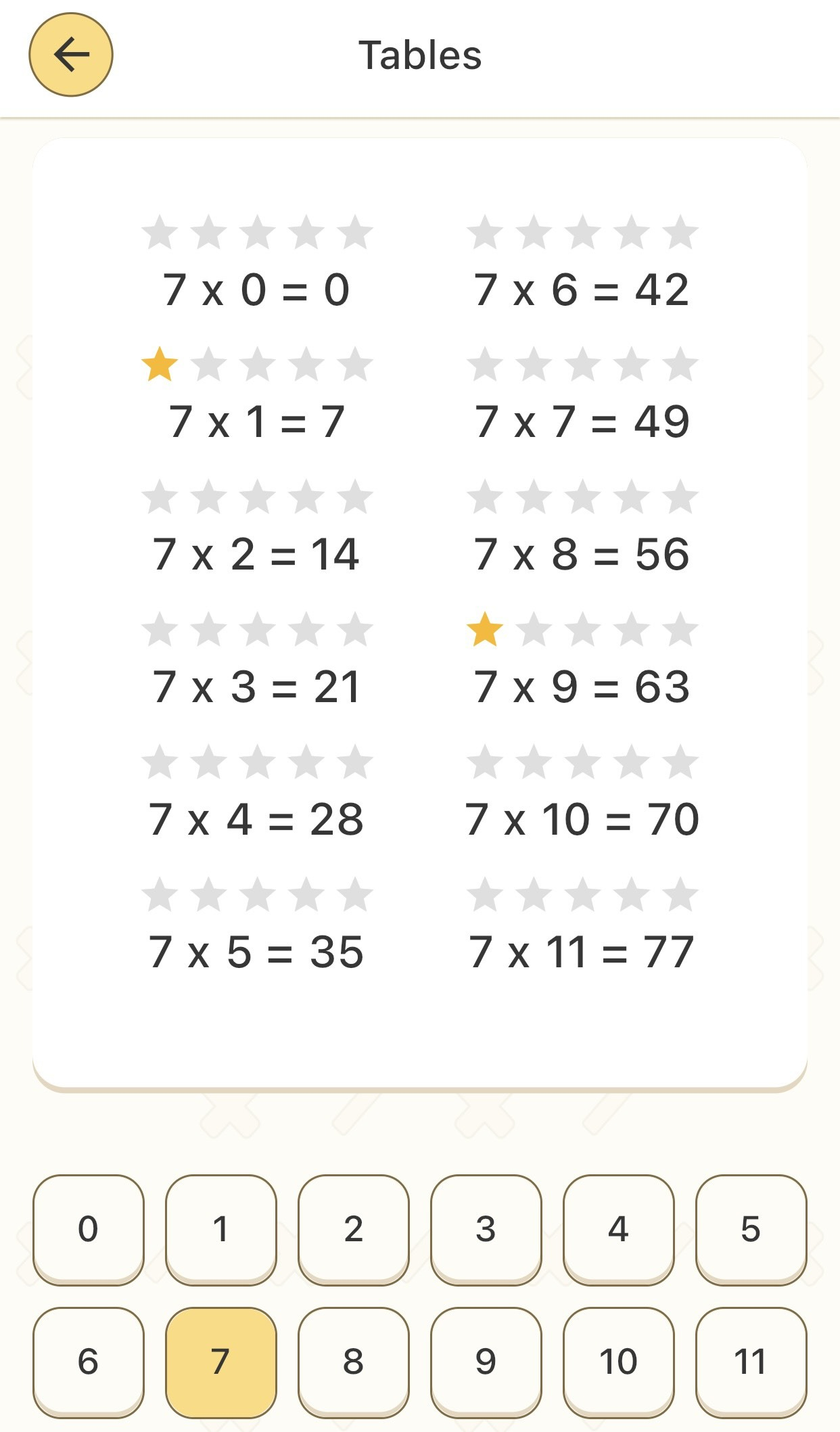# 4 highlighted features of Basic Math

Mathematics is a beneficial and practical subject that shapes children’s cognitive skills, providing a solid foundation for personal development and future success. Basic Math is a mobile math learning application designed to foster mathematical thinking in your child right from the preschool years. Let’s explore the 4 features of Basic Math!

## Features of Basic Math: Tables with Multiplication and Division

The Basic Math app introduces a diverse lesson library, often referred to as the multiplication and division times tables. It focuses on two crucial mathematical concepts: multiplication and division. These lessons are meticulously crafted for clear and straightforward practice, covering multiplication and division of numbers from 0 to 11. This approach helps your child grasp the techniques behind these fundamental operations.Features of Basic Math: Multiplication times table